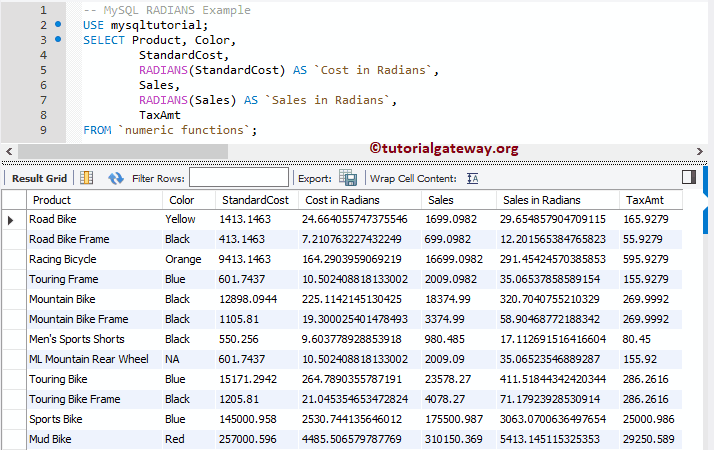MySQL RADIANS function is one of the Numeric Functions, which converts the degree values into radians. The basic syntax of the RADIANS in MySQL is as shown below:

```SELECT RADIANS (Numeric_Expression)
FROM Source```

To demonstrate this RADIANS Numeric function, we are going to use the below shown data

The MySQL RADIANS convert the degree value into radians. The following query shows multiple ways to use the RADIANS function.

```-- MySQL RADIANS Function Example

-- Finding Radians Value for Negative Value

-- Finding Radians Value for Positive Value

-- Finding Radians Value for string value

-- Finding Radians Value of a String

From the below screenshot, you can see that we used the MySQL RADIANS function on different values.

The RADIANS Numeric Function also allows you to find the radian’s value for the column data. In this example, we are going to find the equivalent radians value for all the records present in the Standard Cost and Sales column.

```-- MySQL RADIANS Example
USE mysqltutorial;
SELECT Product, Color,
StandardCost,